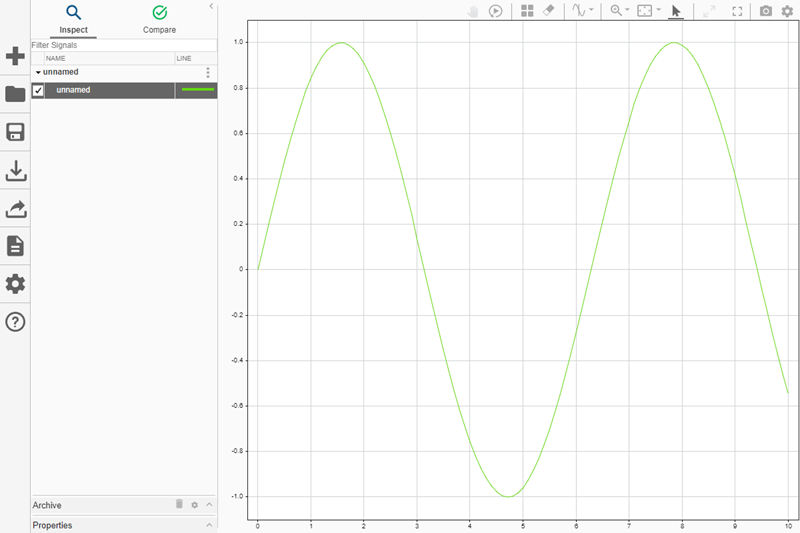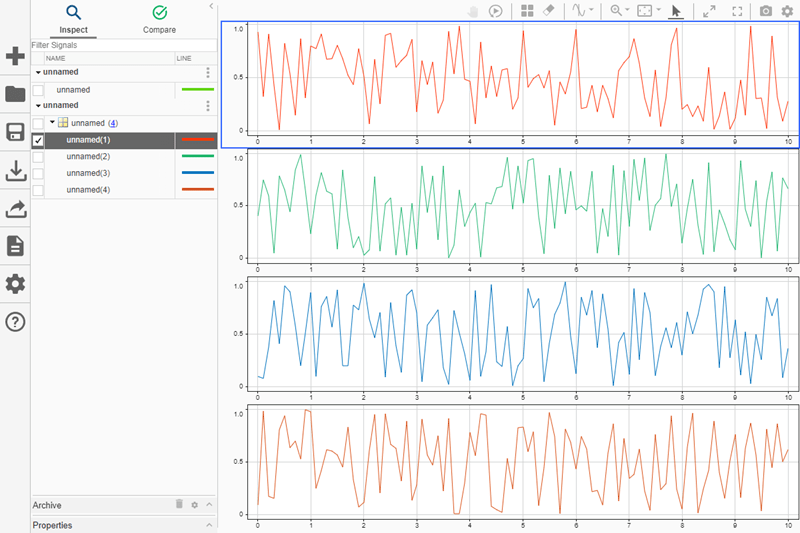Plot data in Simulation Data Inspector

Since R2019b

## Syntax

``Simulink.sdi.plot(obj)``
``Simulink.sdi.plot(obj,runName)``
``Simulink.sdi.plot(data,time)``
``runObj = Simulink.sdi.plot(___)``

## Description

example

````Simulink.sdi.plot(obj)` opens the Simulation Data Inspector and plots the data in the object `obj`.When the input object contains fewer than eight signals, the Simulation Data Inspector layout changes to `1`-by-n, where n is the number of signals, and each subplot displays one signal. When the simulation results contain more than eight signals to plot, the Simulation Data Inspector layout changes to `1`-by-`1`, and the plot displays the first signal. ```
````Simulink.sdi.plot(obj,runName)` names the run `runName` when the data in `obj` does not already exist as a named run in the Simulation Data Inspector. If the data in `obj` already exists as a named run in the Simulation Data Inspector, the run is not renamed.```

example

````Simulink.sdi.plot(data,time)` plots data with respect to time in the Simulation Data Inspector.```
````runObj = Simulink.sdi.plot(___)` returns the `Simulink.sdi.Run` object that corresponds to the plotted data.```

## Examples

collapse all

You can use the `Simulink.sdi.plot` function to plot simulation results in the Simulation Data Inspector. Open the model `vdp`, which models the second-order Van der Pol differential equation. For more information about the model, see Van der Pol Oscillator.

```mdl = "vdp"; open_system(mdl)```Simulate the model. The model logs two signals: `x1` and `x2`. Simulation results are stored in a single `SimulationOutput` object named `out`.

`out = sim(mdl);`

Use the `Simulink.sdi.plot` function to open the Simulation Data Inspector and plot the results.

`Simulink.sdi.plot(out);`You can use the `Simulink.sdi.plot` function to plot data with respect to time in the Simulation Data Inspector.

```time = 0:0.1:10; data = sin(time); Simulink.sdi.plot(data,time);```You can also plot an array of data values in the Simulation Data Inspector. The number of rows in the data array must match the length of the time vector. The number of columns in the data array corresponds to how many signals are plotted.

```dataArray = rand(length(time),4); Simulink.sdi.plot(dataArray,time);```## Input Arguments

collapse all

Data to plot, specified as one of these objects:

When some or all of the data in a `Simulink.SimulationOutput` object is in a Simulation Data Inspector run, the `plot` function opens the Simulation Data Inspector and plots all the signals in the run. When the `SimulationOutput` object does not correspond to a run in the Simulation Data Inspector, the `plot` function imports the data into a new run.

When you use the `plot` function to plot a single signal, the `plot` function always imports the data for the signal into a new run.

Example: `plot(out)` plots the contents of the `Simulink.SimulationOutput` object `out` in the Simulation Data Inspector and opens the Simulation Data Inspector.

Run name, specified as a string or character vector.

The software only applies the run name `runName` to an unnamed run. If a named run with some or all of the data in `obj` already exists in the Simulation Data Inspector, the run is not renamed. When you create a run by simulating a model that logs data, the run name is automatically generated according to the run-naming rule in the Simulation Data Inspector. You can modify the run-naming rule in the Simulation Data Inspector in the UI or by using the `Simulink.sdi.setRunNamingRule` function.

To rename a run, you can use the `Name` property. For example, `myRun.Name = myRunName` sets the `Name` property of the `Run` object `myRun` to `myRunName`. The workspace variable name for the run (`myRun`) does not change when you set the `Name` property.

Sample times, specified as a numeric scalar or vector, or a cell array of date character vectors. The table lists forms for valid date character vectors.

FormatExample
`dd-mmm-yyyy HH:MM:SS``01-Mar-2000 15:45:17`
`dd-mmm-yyyy``01-Mar-2000`
`mm/dd/yy``03/01/00`
`mm/dd``03/01`
`HH:MM:SS``15:45:17`
`HH:MM:SS PM``3:45:17 PM`
`HH:MM``15:45`
`HH:MM PM``3:45 PM`
`mmm.dd,yyyy HH:MM:SS``Mar.01,2000 15:45:17`
`mmm.dd,yyyy``Mar.01,2000`
`mm/dd/yyyy``03/01/2000`

Data Types: `double` | `single` | `int8` | `int16` | `int32` | `int64` | `uint8` | `uint16` | `uint32` | `uint64` | `cell`

Data to plot in the Simulation Data Inspector, specified as a numeric or `logical` scalar, vector, or multidimensional array. The size of the `data` argument must correspond to the size of the `time` argument:

• When `data` is a scalar, `time` must also be a scalar.

• When `data` is a vector, `time` must also be a vector of the same length.

• When `data` is a multidimensional array, `time` must be a vector with a length equal to the number of rows in `data`.

Data Types: `single` | `double` | `int8` | `int16` | `int32` | `int64` | `uint8` | `uint16` | `uint32` | `uint64` | `logical`
Complex Number Support: Yes

## Output Arguments

collapse all

Run that corresponds to plotted data, returned as a `Simulink.sdi.Run` object.

## Version History

Introduced in R2019b